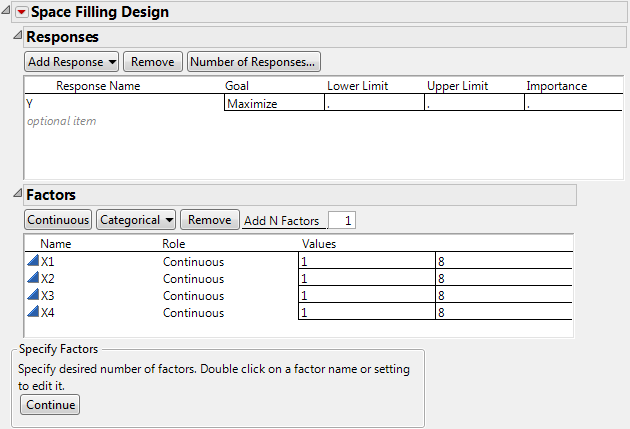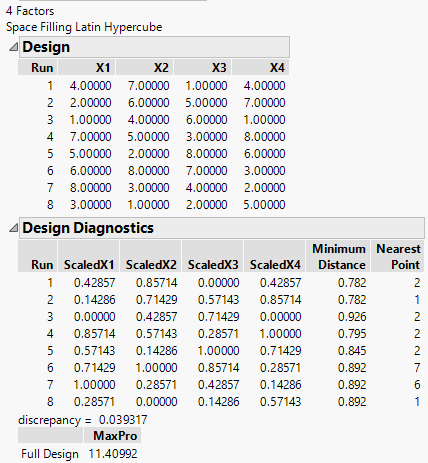Publication date: 11/10/2021

## Creating a Latin Hypercube Design

To create a Latin hypercube design follow these steps:

1. Select DOE > Special Purpose > Space Filling Design.

2. Enter responses, if necessary, and factors.

See Responses.

3. Alter the factor level values, if necessary. Figure 21.10 shows adding four factors and changing their values to 1 and 8 instead of the default –1 and 1.

Figure 21.10 Space-Filling Dialog for Four Factors4. Click Continue.

5. In the design specification dialog, specify a sample size (Number of Runs). This example uses a sample size of eight.

6. Click Latin Hypercube (Figure 21.7). Factor settings and design diagnostics results appear similar to those in Figure 21.11, which shows the Latin Hypercube design with four factors and eight runs.

Note: The purpose of this example is to show that each column (factor) is assigned each level only once, and each column is a different permutation of the levels.

Figure 21.11 Latin Hypercube Design for Four Factors and Eight Runs with Eight Levels